Register for Maths, Science, English, Reasoning Olympiad Exams Register here | Book Free Demo for Live Olympiad Classes here | Check Olympiad Exam Dates here | Buy Practice Papers for IMO, IOM, HEO, IOEL etc here | Login here to participate in all India free mock test on every Saturday

# Temperature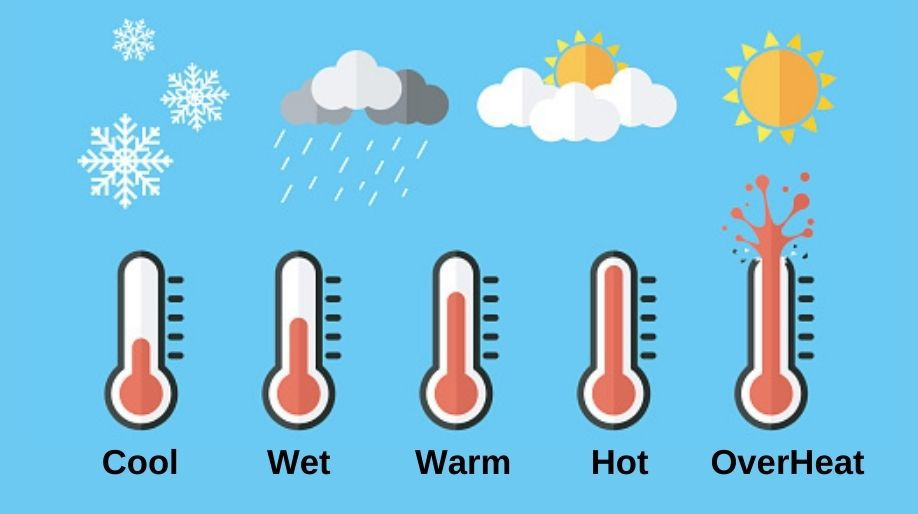What is Temperature? In our everyday life we all listen to a statement “Today the temperature is 23 degree Celsius or 23°C or some other value". Now have you ever asked yourself a question what exactly does temperature means? Let us understand it.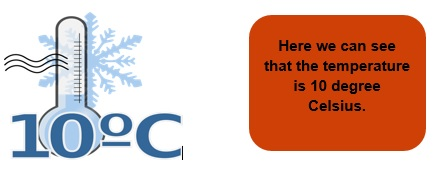• The intensity of the heat present in any body is known as temperature.

• It is measured using an instrument called Thermometer.
• The unit in which it is measured is Degree Celsius(°C) and the standard unit is Kelvin.(K)

Units and Conversions

There different units to measure the temperature and are listed below:

1. Degree Centigrade or Degree Celsius – written as °C.
2. Degree Fahrenheit - written as °F.
3. Kelvin- written as K.

Kelvin is considered as the SI unit of temperature.

Formula:

 Temperature in degree Celsius = 273 - Temperature in Kelvin

Example 1: Convert 23°C into Kelvin.

Solution: 1°C = 273- Temperature in K

Therefore 1 Kelvin = 273 – 1°C = 272 K.

Conversion from Degree Celsius to Fahrenheit:

The conversion can be done in following 3 steps:

• Multiply the temperature in degree with 9.
• Divide the answer by 5.

Example 2: Convert 23°C to °F.

Solution: Following the above 3 steps one by one we get,

(23 x 9) = 207 and then

(207 ÷ 5) = 41.4 and then

41.4 + 32 = 71.4°F.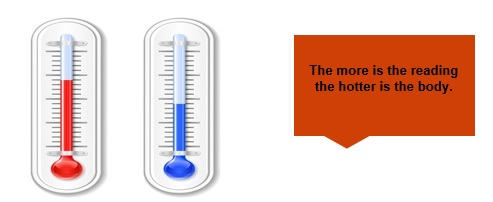From this figure we can learn two things:

• As the reading of thermometer increases the value of heat increases and hence the body becomes more hot.
• As the reading of thermometer decreases the value of heat decreases and hence the body becomes more cold.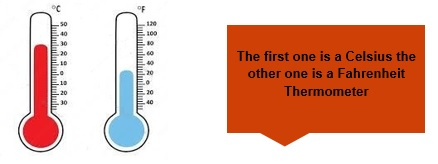Points to Remember

• The normal temperature of a human body is 37 degree Celsius.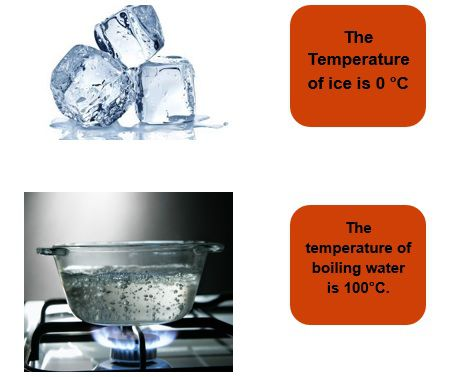• The Celsius is marked from 0°C to 100°C.
• The Fahrenheit scale is marked from 32°F to 212°F.
• The thermometer used for the medical purpose has a special name Clinical Thermometer.

Practice Question

Q1) What is the normal temperature of a human body?

Q2) At which temperature water starts to boil?

Q3) Convert the following to Degree Fahrenheit.

1. 0°C
2. 100°C
3. 37°C

Q4) State True or False:

1. 1°C = 273 K
2. Water freezes at 100°C.
3. Temperature of ice is 0°C.

Recap

• Thermometer is used to measure temperature of any body.
• Unit of temperature can be K or °C or °F.
• Temperature in °C = 273 – Temperature in K.
• 0°C = 32°F.
• Temperature of ice is 0°C or 32°F.
• Doctors use Clinical Thermometer to measure temperature of patients.

## Quiz for Temperature

 Q.1 Convert 41 °F into °C. a) 5 °C b) 10 °C c) 15 °C d) -5 °C
 Q.2 Convert 68 °F into °C. a) 5 °C b) 10 °C c) -20 °C d) 20 °C
 Q.3 Water freezes at ________. a) 100 °C b) - 1 °C c) 0 °C d) -10 °C
 Q.4 What is the addition of temperature 4 °C and 73 °C? a) 77 °C b) 69 °C c) 75 °C d) none of the above
 Q.5 Convert temperature from Celsius to Fahrenheit: 32 °C a) 80 °F b) 90 °F c) 90.6 °F d) 89.6 °F
 Q.6 Convert temperature from Celsius to Fahrenheit: 6 °C a) 6 °F b) 32 °F c) 44 °F d) 42.8 °F
 Q.7 What is the addition of temperature 8 °C and -3 °C? a) 11 °C b) 4 °C c) 5 °C d) 6 °C
 Q.8 The daytime temperature is 12 °C, but by evening it has fallen by 4 degrees. What is the temperature in the evening? a) 9 °C b) 8 °C c) 7 °C d) 16 °C
 Q.9 Convert 36 °C into K. a) 309.15 K b) 360 K c) 237.15 K d) none of the above
 Q.10 You decide to wear a jacket to go outside your house. What is the temperature most likely to be? a) 68 °C b) 20 °C c) 40 °C d) 45 °C